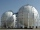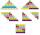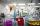# Hollow sphere

The volume of the hollow ball is 3432 cm3. What is its internal radius when the wall thickness is 3 cm?

Result

r =  8 cm

#### Solution:Checkout calculation with our calculator of quadratic equations.

Leave us a comment of example and its solution (i.e. if it is still somewhat unclear...):

Showing 0 comments:Be the first to comment!#### To solve this example are needed these knowledge from mathematics:

Do you have a linear equation or system of equations and looking for its solution? Or do you have quadratic equation?

## Next similar examples:

1. Hemispherical hollowThe vessel hemispherical hollow is filled with water to a height of 10 cm =. How many liters of water are inside if the inside diameter of the hollow is d = 28cm?
2. Spherical tankThe tank of a water tower is a sphere of radius 35ft. If the tank is filled to one quarter of full, what is the height of the water?
3. Cube cornersFrom cube of edge 14 cm cut off all vertices so that each cutting plane intersects the edges 1 cm from the nearest vertice. How many edges will have this body?
4. TetrahedronCalculate height and volume of a regular tetrahedron whose edge has a length 18 cm.
5. Holidays - on poolChildren's tickets to the swimming pool stands x € for an adult is € 2 more expensive. There was m children in the swimming pool and adults three times less. How many euros make treasurer for pool entry?
6. Theorem proveWe want to prove the sentense: If the natural number n is divisible by six, then n is divisible by three. From what assumption we started?
7. Square root 2If the square root of 3m2 +22 and -x = 0, and x=7, what is m?
8. Fifth memberDetermine the fifth member of the arithmetic progression, if the sum of the second and fifth members equal to 73, and difference d = 7.
9. Determine APDetermine the difference of the arithmetic progression if a3 = 7, and a4 + a5 = 71
10. EquationHow many real roots has equation ? ?
11. Expression with powersIf x-1/x=5, find the value of x4+1/x4
12. AlgebraX+y=5, find xy (find the product of x and y if x+y = 5)
13. Crystal waterThe chemist wanted to check the content of water of crystallization of chromic potassium alum K2SO4 * Cr2 (SO4) 3 * 24 H2O, which was a long time in the laboratory. From 96.8 g of K2SO4 * Cr2 (SO4) 3 * 24 H2O prepared 979 cm3 solution of base. S
14. Difference AP 4Calculate the difference of the AP if a1 = 0.5, a2 + a3 = -1.1
15. Evaluation of expressionsIf a2-3a+1=0, find (i)a2+1/a2 (ii) a3+1/a3
16. CoefficientDetermine the coefficient of this sequence: 7.2; 2.4; 0.8
17. ChildrenThe group has 42 children. There are 4 more boys than girls. How many boys and girls are in the group?